31.6 C
Jaipur
Wednesday, June 16, 2021

# Setting Decimal Precision in C Language – Linux Hint

### 5 best Father’s Day gift ideas under Rs 2000 for your tech savvy dad

This article will show you how to set decimal precision in C programming language. First, we will define precision, and then, we will look into multiple examples to show how to set decimal precision in C programming.

## Decimal Precision in C

The integer type variable is normally used to hold the whole number and float type variable to hold the real numbers with fractional parts, for example, 2.449561 or -1.0587. Precision determines the accuracy of the real numbers and is denoted by the dot (.) symbol. The Exactness or Accuracy of real numbers is indicated by the number of digits after the decimal point. So, precision means the number of digits mentioned after the decimal point in the float number. For example, the number 2.449561 has precision six, and -1.058 has precision three.

As per IEEE-754 single-precision floating point representation, there are a total of 32 bits to store the real number. Of the 32 bits, the most significant bit is used as a sign bit, the following 8 bits are used as an exponent, and the following 23 bits are used as a fraction.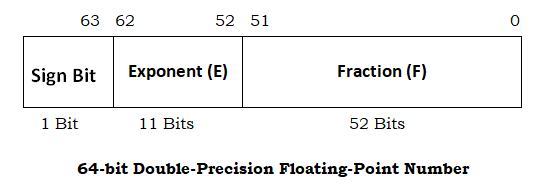In the case of IEEE-754 double-precision floating point representation, there are a total of 64 bits to store the real number. Of the 64 bits, the most significant bit is used as a sign bit, the following 11 bits are used as an exponent, and the following 52 bits are used as a fraction.

However, when printing the real numbers, it is necessary to specify the precision (in other words, accuracy) of the real number. If the precision is not specified, the default precision will be considered, i.e., six decimal digits after the decimal point. In the following examples, we will show you how to specify precision when printing floating-point numbers in the C programming language.

## Examples

Now that you have a basic understanding of precision, let us look at a couple of examples:

1. Default precision for float
2. Default precision for double
3. Set precision for float
4. Set precision for double

## Example 1: Default Precision for Float

This example shows that the default precision is set to six digits after the decimal point. We have initialized a float variable with the value 2.7 and printed it without explicitly specifying the precision.

In this case, the default precision setting will ensure that six digits after the decimal point are printed.

#include <stdio.h>

int main()
{
float f = 2.7;

printf(nValue of f     =  %f n, f);
printf(“Size of float    =  %ld n, sizeof(float));

return 0;
}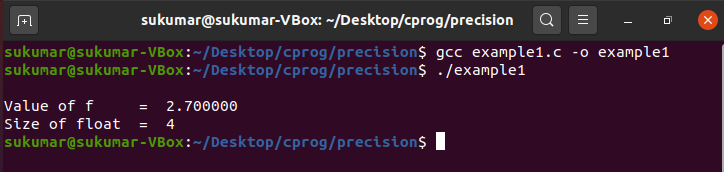## Example 2: Default Precision for Double

In this example, you will see that the default precision is set to six digits after the decimal point for double type variables. We have initialized a double variable, i.e., d, with the value 2.7 and printed it without specifying the precision. In this case, the default precision setting will ensure that six digits after the decimal point are printed.

#include <stdio.h>

int main()
{
double d = 2.7;

printf(nValue of d      =  %lf n, d);
printf(“Size of double  =  %ld n, sizeof(double));

return 0;
}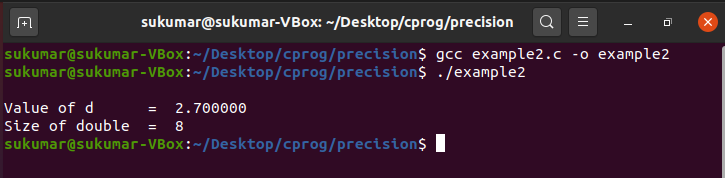## Example 3: Set Precision for Float

Now, we will show you how to set precision for float values. We have initialized a float variable, i.e., f, with the value 2.7, and printed it with various precision settings. When we mention “%0.4f” in the printf statement, this indicates that we are interested in printing four digits after the decimal point.

#include <stdio.h>

int main()
{
float f = 2.7;

/* set precision for float variable */
printf(nValue of f (precision  = 0.1)     =  %0.1f n, f);
printf(nValue of f (precision  = 0.2)     =  %0.2f n, f);
printf(nValue of f (precision  = 0.3)     =  %0.3f n, f);
printf(nValue of f (precision  = 0.4)     =  %0.4f n, f);

printf(nValue of f (precision  = 0.22)    =  %0.22f n, f);
printf(nValue of f (precision  = 0.23)    =  %0.23f n, f);
printf(nValue of f (precision  = 0.24)    =  %0.24f n, f);
printf(nValue of f (precision  = 0.25)    =  %0.25f n, f);
printf(nValue of f (precision  = 0.40)    =  %0.40f n, f);

printf(“Size of float  =  %ld n, sizeof(float));

return 0;
}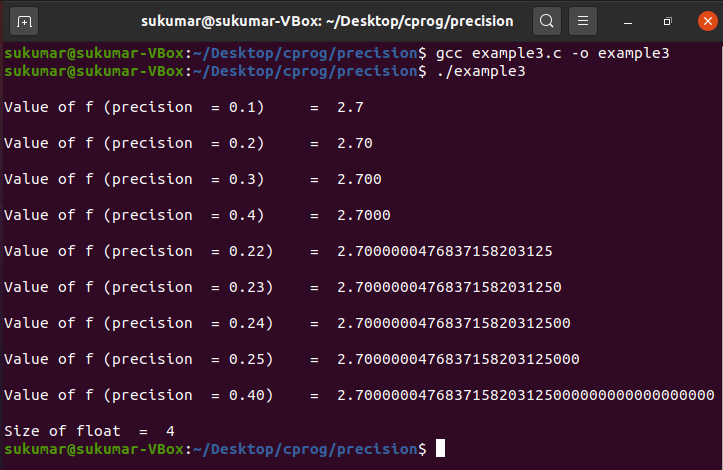## Example 4: Set Precision for Double

In this example, we will see how to set precision for double values. We have initialized a double variable, i.e., d, with the value 2.7 and printed it with various precision settings. When we mention “%0.52f” in the printf statement, this indicates that we are interested in printing 52 digits after the decimal point.

#include <stdio.h>

int main()
{
float f = 2.7;

/* set precision for float variable */
printf(nValue of f (precision  = 0.1)     =  %0.1f n, f);
printf(nValue of f (precision  = 0.2)     =  %0.2f n, f);
printf(nValue of f (precision  = 0.3)     =  %0.3f n, f);
printf(nValue of f (precision  = 0.4)     =  %0.4f n, f);

printf(nValue of f (precision  = 0.22)    =  %0.22f n, f);
printf(nValue of f (precision  = 0.23)    =  %0.23f n, f);
printf(nValue of f (precision  = 0.24)    =  %0.24f n, f);
printf(nValue of f (precision  = 0.25)    =  %0.25f n, f);
printf(nValue of f (precision  = 0.40)    =  %0.40f n, f);

printf(“Size of float  =  %ld n, sizeof(float));

return 0;
}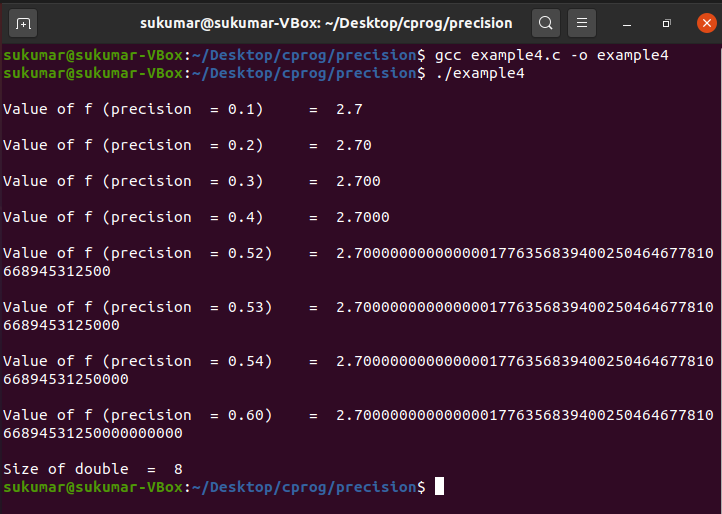## Conclusion

Precision is a very important factor for representing a real number with adequate accuracy. The c programming language provides the mechanism to control the accuracy or exactness of a real number. However, we cannot change the actual precision of the real number. For example, the fraction part of a 32-bit single-precision floating-point number is represented by 23 bits, and this is fixed; we cannot change this for a particular system. We can only decide how much accuracy we want by setting the desired precision of the real number. If we need more accuracy, we can always use the 64-bit double-precision floating-point number.

Source link

- Advertisement -#### More articles

- Advertisement -